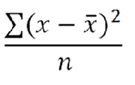引用：基本函数

绝对值 (Row)

ABS(metric)

metric 您希望求绝对值的量度。

MAXV(metric)

metric 您希望计算的量度。

MINV(metric)

metric 您希望计算的量度。

列总和

SUM(metric)

metric 您希望求总值或总和的量度。

COUNT(metric)

metric 您希望计数的量度。

指数 (Row)

EXP(metric)

metric 应用于底数 e 的指数。

求幂

pow(x,y) = x<sup>y</sup> = x*x*x*… (y times)

平均值 (Table)

MEAN(metric)

metric 您希望求平均数的量度。

中间值 (Table)

MEDIAN(metric)

metric 您希望求中间值的量度。

取模

x = floor(x/y) + modulo(x,y)

modulo(4,3) = 1
modulo(-4,3) = -1
modulo(-3,3) = 0

modulo(modulo(x,y)+y,y)

百分位数 (Table)

PERCENTILE(metric,k)

metric 用于定义相对位置的量度列。
k 介于 0 到 100 之间（包括 0 和 100）的百分位数值。

四分位数 (Table)

QUARTILE(metric,quart)

metric 您希望求四分位数值的量度。
quart 指示要返回哪个*值。

*如果 quart = 0，则 QUARTILE 返回最小值。如果 quart = 1，则 QUARTILE 返回第一个四分位数（第 25 个百分位数）。如果 quart = 2，则 QUARTILE 返回第一个四分位数（第 50 个百分位数）。如果 quart = 3，则 QUARTILE 返回第一个四分位数（第 75 个百分位数）。如果 quart = 4，则 QUARTILE 返回最大值。

轮次

ROUND(metric)

round( 314.15, 0) = 314
round( 314.15, 1) = 314.1
round( 314.15, -1) = 310
round( 314.15, -2) = 300

SQRT(metric)

标准偏差 (Table)

STDEV 的方程式为：STDEV(metric)

metric 您希望求标准偏差的量度。

变量 (Table)

VARIANCE 的方程式为：VARIANCE(metric)

metric 您希望求变量的量度。

1. 从数字中减去平均值。
2. 将结果求平方。
3. 再相加求和。

1

2

3